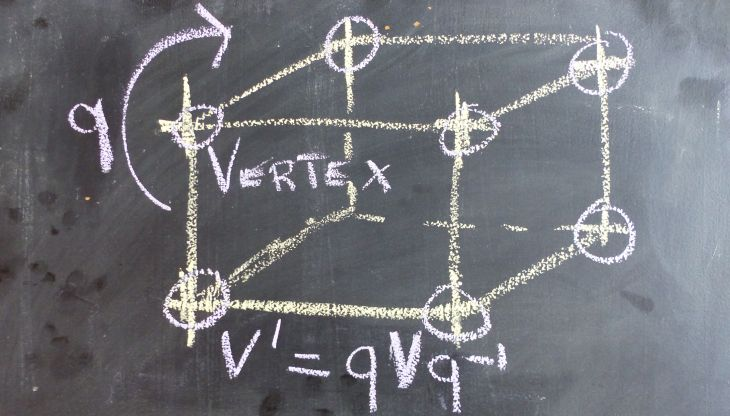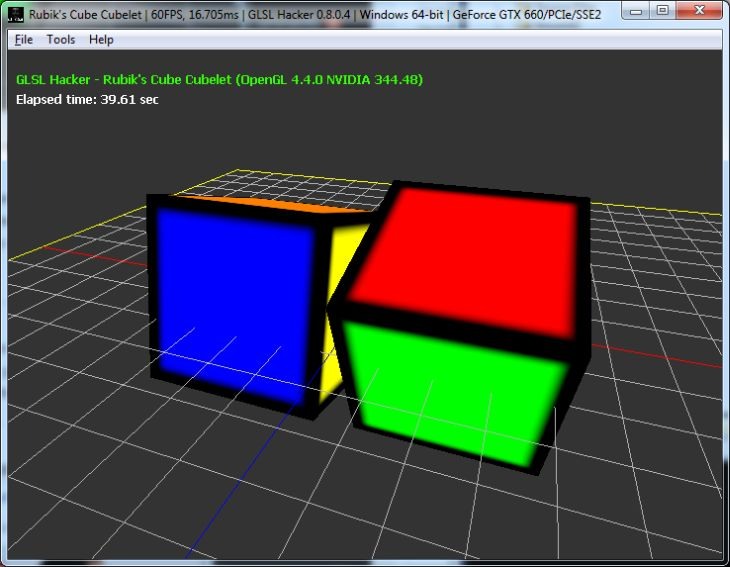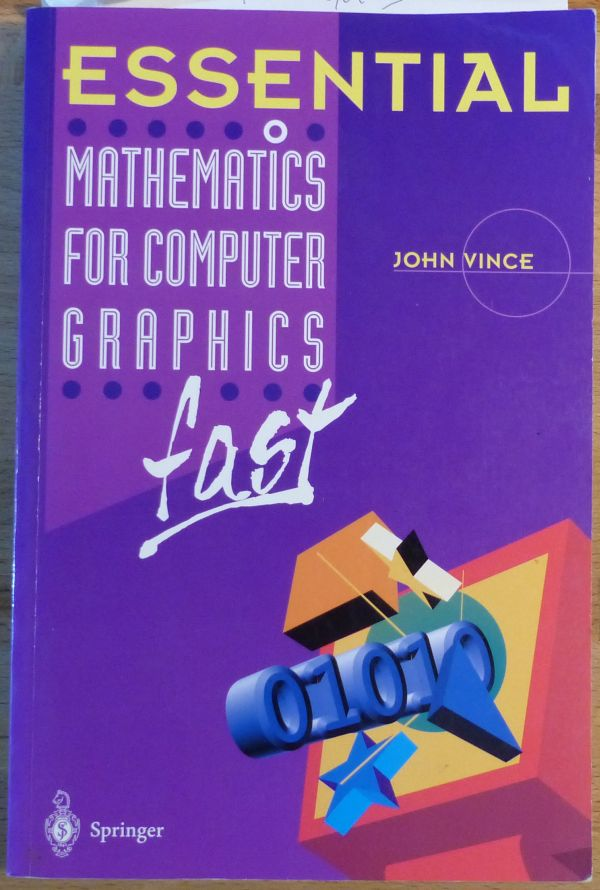# How to Rotate a Vertex by a Quaternion in GLSL (*Updated*)In a vertex shader, the rotation and position are usually encoded in the model matrix and we have something like this:

```vec4 worldPos = ModelMatrix * InPosition;
```

Here is another method to transform the position of a vertex, using a quaternion to hold the rotation information. Quaternions are a fantastic mathematics tool discovered by Sir William Rowan Hamilton in 1843. We’re not going to review quaternions in detail here, because I’m not a mathematician and it’s not the point. We’re going to see how to use them in practice in a GLSL program to rotate a vertex.

A quaternion can be seen as a object that holds a rotation around any axis. A quaternion is a 4D object defined as follows:

```q = [s, v]
q = [s + xi + yj + zk]
```

where s, x, y and z are real numbers. s is called the scalar part while x, y and z form the vector part. i, j and k are imaginary numbers. Quaternions are the generalization of complex numbers in higher dimensions.

In 3D programming, we store quaternions in a 4D vector:

```q = [x, y, z, w]
```

where w = s and [x, y, z] = v.

Now let’s see the fundamental relation that makes it possible to rotate a point P0 around an rotation axis encoded in the quaternion q:

```P1 = q P0 q-1
```

where P1 is the rotated point and q-1 is the inverse of the quaternion q.

From this relation we need to know:
1 – how to transform a rotation axis into a quaternion.
2 – how to transform a position into a quaternion.
3 – how to get the inverse of a quaternion.
4 – how to multiply two quaternions.

Remark: all the following rules expect an unit quaternion. An unit quaternion is a quaternion with a norm of 1.0. A quaternion can be normalized with:

```norm = sqrt(q.x*q.x + q.y*q.y + q.z*q.z + q.w*q.w)
q.x =  q.x / norm
q.y =  q.y / norm
q.z =  q.z / norm
q.w =  q.w / norm
```

## 1 – How to transform a rotation axis into a quaternion

Here is a formula that converts a rotation around an axis (defined by the couple [axis, angle]) into a quaternion:

```half_angle = angle/2
q.x = axis.x * sin(half_angle)
q.y = axis.y * sin(half_angle)
q.z = axis.z * sin(half_angle)
q.w = cos(half_angle)
```

## 2 – How to transform a position into a quaternion

The position is usually a 3D vector: {x, y, z}. This position can be represented in a quaternion by setting to zero the scalar part and initializing the vector part with the xyz-position:

```q.x = position.x
q.y = position.y
q.z = position.z
q.w = 0
```

The quaternion q=[x, y, z, 0] is a pure quaternion because it has not real part.

## 3 – How to get the inverse of a quaternion

The inverse of a quaternion is defined by the following relation:

```q = [x, y, z, w]
norm = |q| = sqrt(q.x*q.x + q.y*q.y + q.z*q.z + q.w*q.w)
q-1 = [-x, -y, -z, w] / |q|
q-1 = [-x/|q|, -y/|q|, -z/|q|, w/|q|]
```

If we have an unit quaternion, |q|=1 and the inverse is equal to the conjugate (q*) of the quaternion:

```q-1 = q* = [-x, -y, -z, w]
```

## 4 – How to multiply two quaternions

Quaternions can be multiplied:

```q = q1 * q2
```

But like for matrix multiplication, quaternion multiplication is non-commutative:

```(q1 * q2) != (q2 * q1)
```

The multiplication of two quaternions is defined by:

```q.x = (q1.w * q2.x) + (q1.x * q2.w) + (q1.y * q2.z) - (q1.z * q2.y)
q.y = (q1.w * q2.y) - (q1.x * q2.z) + (q1.y * q2.w) + (q1.z * q2.x)
q.z = (q1.w * q2.z) + (q1.x * q2.y) - (q1.y * q2.x) + (q1.z * q2.w)
q.w = (q1.w * q2.w) - (q1.x * q2.x) - (q1.y * q2.y) - (q1.z * q2.z)
```

Now we have all tools to rotate a point around an axis in a GLSL vertex shader:

```#version 150
in vec4 gxl3d_Position;
in vec4 gxl3d_TexCoord0;
in vec4 gxl3d_Color;
out vec4 Vertex_UV;
out vec4 Vertex_Color;
uniform mat4 gxl3d_ViewProjectionMatrix;

struct Transform
{
vec4 position;
vec4 axis_angle;
};
uniform Transform T;

vec4 quat_from_axis_angle(vec3 axis, float angle)
{
vec4 qr;
float half_angle = (angle * 0.5) * 3.14159 / 180.0;
qr.x = axis.x * sin(half_angle);
qr.y = axis.y * sin(half_angle);
qr.z = axis.z * sin(half_angle);
qr.w = cos(half_angle);
return qr;
}

vec4 quat_conj(vec4 q)
{
return vec4(-q.x, -q.y, -q.z, q.w);
}

vec4 quat_mult(vec4 q1, vec4 q2)
{
vec4 qr;
qr.x = (q1.w * q2.x) + (q1.x * q2.w) + (q1.y * q2.z) - (q1.z * q2.y);
qr.y = (q1.w * q2.y) - (q1.x * q2.z) + (q1.y * q2.w) + (q1.z * q2.x);
qr.z = (q1.w * q2.z) + (q1.x * q2.y) - (q1.y * q2.x) + (q1.z * q2.w);
qr.w = (q1.w * q2.w) - (q1.x * q2.x) - (q1.y * q2.y) - (q1.z * q2.z);
return qr;
}

vec3 rotate_vertex_position(vec3 position, vec3 axis, float angle)
{
vec4 qr = quat_from_axis_angle(axis, angle);
vec4 qr_conj = quat_conj(qr);
vec4 q_pos = vec4(position.x, position.y, position.z, 0);

vec4 q_tmp = quat_mult(qr, q_pos);
qr = quat_mult(q_tmp, qr_conj);

return vec3(qr.x, qr.y, qr.z);
}

void main()
{
vec3 P = rotate_vertex_position(gxl3d_Position.xyz, T.axis_angle.xyz, T.axis_angle.w);
P += T.position.xyz;
gl_Position = gxl3d_ViewProjectionMatrix * vec4(P, 1);
Vertex_UV = gxl3d_TexCoord0;
Vertex_Color = gxl3d_Color;
}
```

Update (2014.12.02): the rotate_vertex_position() function can be optimized a little bit with:

```vec3 rotate_vertex_position(vec3 position, vec3 axis, float angle)
{
vec4 q = quat_from_axis_angle(axis, angle);
vec3 v = position.xyz;
return v + 2.0 * cross(q.xyz, cross(q.xyz, v) + q.w * v);
}
```

This powerful vertex shader comes from the host_api/RubikCube/Cube_Rotation_Quaternion/demo_v3.xml demo you can find in the code sample pack. To play with this demo, GLSL Hacker v0.8+ is required.Some references:## 4 thoughts on “How to Rotate a Vertex by a Quaternion in GLSL (*Updated*)”

1.Kristine

This strikes me as highly complicated and inefficient compared to (q is the quaternion, v the vector to rotate):

return v + 2.0 * cross(q.xyz, cross(q.xyz, v) + q.w * v);

2.JeGX Post Author

Yes you’re right, the code is not optimized, but I wanted to detail this popiular method to rotate a vector (qPq-1). I updated the article with your code. Thanks!

3.Reza Nezami

With respect to the optimized version of vertex rotation, I suggest keep the original one and provide the optimized version as what it is,i.e. an optimization. The code you have provided above, as I understand, is mostly for educational purpose and your original method was educational, but the optimized method is really not! I mean first it must be shown how this is equivalent to the original version, if you really want to replace yours with the more efficient one! Good job.

4.Kristine

@Reza: I agree with you. Also when it comes to books I like “Visualizing Quaternions” by Andrew Hanson.